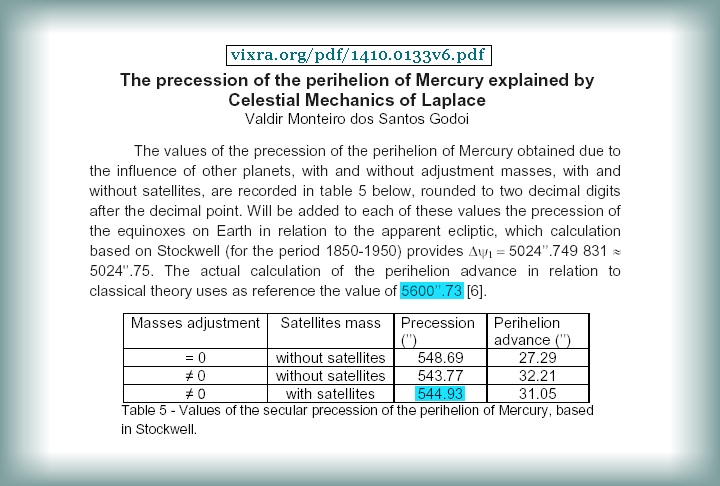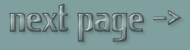Perihelion Precession and N-body-Gravity

 Perihelion Precession is how the extremity of an orbit changes position between orbits. However, Perihelion Precession is not at all constant as is commonly described. The extremities of an orbit (major axis) vary from orbit to orbit by moving in both directions considerably, depending on which orbit is being noted. This occurs due to the gravity of the other planets, which then shift both the perihelion and the aphelion behind or ahead; marginally distorting its expected elliptical position. This is obviously dependant on the other planet's exact position in comparison to the planet being examined. For Mars especially, orbits must be grouped in cycles of at least 59 years to average out the various effects of Jupiter and Saturn. To take a 100 year sample would prove to be less accurate than an average that uses a 59 year sample. (In the section Jupiter+Saturn, there is more detail.) But for Mercury we need to measure in batches of 492 orbits to balance the varying proportional effects of Jupiter and Saturn with Venus. This would be 118.5 Earth-years; so we need to double that amount again to keep Mercury's orbit in synch with Earth's orbit. So it is even more accurate to measure Mercury's Perihelion Precession in batches of 237 Earth-orbits which is 984 Mercury-orbits - and this is fairly close to 3 orbits of Uranus! But the highest ideal measurement for the Jupiter-Saturn interaction is 913 years. Because that represents a very good ratio of 31 to 77 orbits respectively. And that is near to Uranus orbiting 11 times. Even though most orbits precede (or advance) the major axis on average, some orbits recede the Perihelion and Aphelion, often by amounts numerous times greater than the average. And such detail cannot be noticed using the numerical models for Perihelion Precession which have been detailed historically. And this is how the 3d-n-body-gravity model improves on numerical methods. Those numerical methods add 7 approximate 2D influences of the other planets to Mercury's orbit in addition to the gravity of the Sun. But OGS15 (orbit-gravity-sim-15.exe) takes into account all 72 detailed interactions between the 9 primary bodies in 3D.So how accurate is the ring-planet model? (diagram above) There is a fundamental difference in process as to how the algorithm OGS15 (orbit-gravity-sim-15.exe) works when compared to this. The numerical models add up the various effects of each planet using quite complex mathematical approximations, whereas OGS15 just uses just the formula of g=Gm/r^2 from Newton as well as the notion of quantum-time from Planck. So OGS15 is a 3D evolutionary model whereby each planet is constantly altering every other planet. It is conceptually simpler, and yet effectively more accurate because it takes into account more interactions. Of course the computer does all the arithmetic for us: Countless billions of simple calculations, with each iota of time accounted for; and this is something impossible for humans to deal with directly using pencil-paper math. OGS15 does have a flaw in that the time-quanta it uses cannot possibly be close to Planck-time because we do not have computers that can process anywhere near that rate of 5.4 x 10^-44 seconds per calculation. In the same context, every moon is not calculated directly. The masses of the moons have simply been added to the mass of the parent body. Adding the data of each little moon separately would not be a problem for the algorithm, but it would slow the process down and thus be less accurate. So the flaw is not in the algorithm, but in the accuracy which can be attained by the processing power of the computer it is running on. But these error-margins are mostly insignificant, as will be shown later. The computer used to construct OGS15 is an ordinary 2014 entry level IBM machine (later inadvertently down-graded to a 2017 Windows 10 contraption - with 4 lazy cores). But the algorithm itself can operate more accurately when run on faster machines. So you, the user, might likely get better results from my software than I can, without doing anything much more than clicking the start button, then observing the results after the algorithm has evolved. Thus the methods used historically to calculate Perihelion Precession between orbits are astonishingly crude by computational standards. Even then, they are actually more complex than OGS15 from a mathematical perspective. But all of the numerical models I have seen are merely in 2D, whereas OGS15 is 3-dimensional. However, I also use algorithm OGS13 which is in 2D, for vital comparative purposes. The famous Horizon Ephemeris uses excellent 3D observational data, but it predicts each planet's future movements individually using what I can only figure as being statistical and/or numerical methods. Horizon Ephemeris is thus not a genuine n-body-gravity evolutionary model. (I'll prove this claim later.) Here is another account of Mercury's Perihelion Precession:Godoi at least has noted the classical logarithmic disagreement. Though this quote leaves it unaccounted for. So far we have seen claims that Newton's formula gives Mercury's Perihelion Precession as a change of arc-seconds per Earth-year being theoretically:
5.5
, or 5.3~, or 5.45, and 55.57!

And the observations are said to be: 5.75 and 56.00! per year of Earth. Of course one of those is at least misleading, but there is more groundwork to be covered before we can see if such disagreement is real or apparent. And this involves another similar sounding, but entirely different celestial movement, as mentioned in the quote above, and this is called the Precession of the Equinoxes.

 see also: — details of Mercury's orbit — Mercury's orbit & RelativitySections of this Article by web-page N-body-gravity Algorithm Introduction Mercury Perihelion - Perihelion Precession - Precession of the Equinox Mercury - Venus - Earth - Mars - Jupiter - Saturn - Uranus - Neptune Jupiter+Saturn - Mars+Jupiter+Earth - Orrery Download - How to Build N-body-gravity Algorithm - Sorting Horizons Newtonian-Planck Gravity - Sidereal Year or Tropical Year Cause of Precession of the Equinox - The Scientific Method Discussion Forum : cosmology.africamotion.net page 3